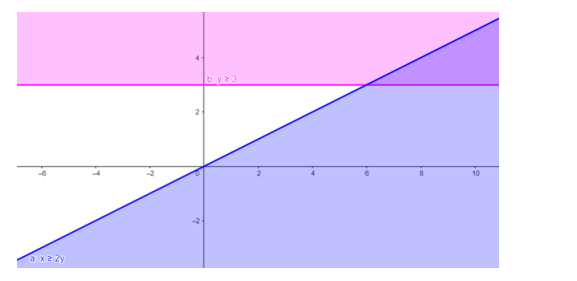# Solve the given inequalities

Question:

Solve the given inequalities $x \geq 2 y, y \geq 3$ graphically in two-dimensional plane.

Solution:

The graphical representation of $x \geq 2 y, y \geq 3$ is given by common region in the figure below.

$x \geq 2 y \ldots \ldots(1)$

$y \geq 3 \ldots \ldots(2)$

Inequality (1) represents the region below line $x=2 y$ (including the line $x=2 y$ ).

Inequality $(2)$ represents the region above line $y=3($ including the line $y=3)$.

Therefore, every point in the common shaded region including the points on the respective lines represents the solution for the given inequalities.

This can be represented as follows,